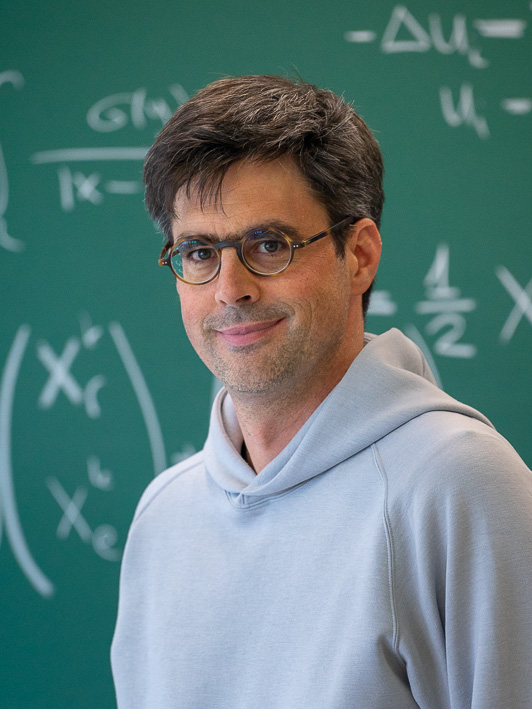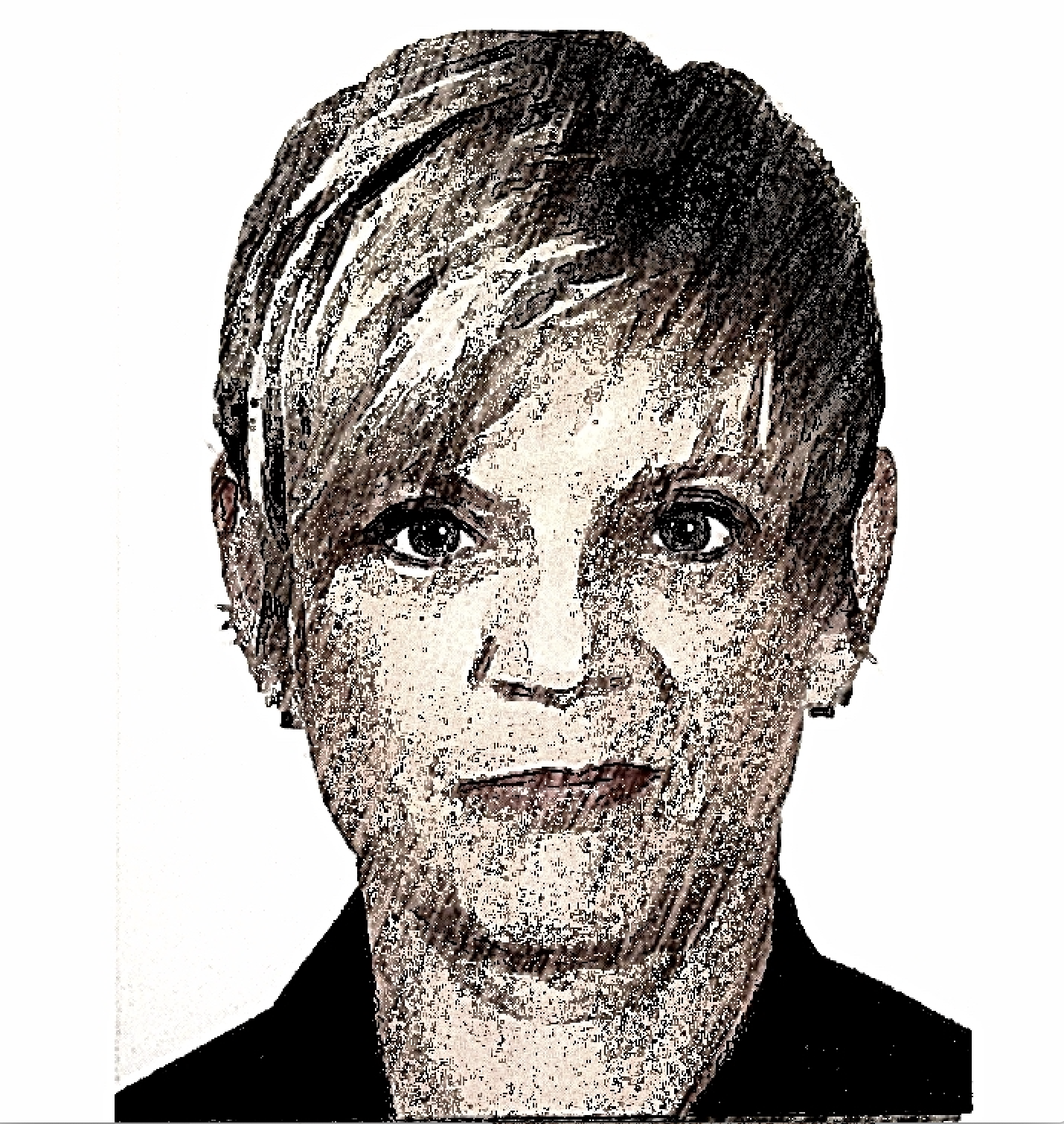# Research

Details about our current projects and research topics (Pages are under construction)

## Scientific interests

Numerical analysis, scientific computing and simulations and in particular:

• Efficient discretizations and solvers for partial differential equations (PDE's) and eigenvalue problems
• Error certification and aposteriori error estimates
• Reduced order modeling
• High Performance Computing (HPC) implementations
• Linear and nonlinear eigenvalue problems in quantum mechanics
• Modeling, analysis and simulation of atomistic models
• Implicit solvation models in computational chemistry
• Electronic structure calculations and density functional theory (DFT)
• Polarizable force-fields
• Electrostatic interaction of dielectric particles
• Stochastic homogenization
• Electromagnetic scattering

## Third-party funded project, Cluster of Excellence EXC 2075, "Data-Integrated Simulation Science (SimTech)"

In this project, we investigate the manipulation, approximation, and learning of density matrices arising in electronic structure calculation in computational chemistry, material science, and solid-state physics. Electronic structure calculation aims at finding the state of the electrons of a given molecular system specified by the nuclear coordinates. The density matrix can be seen as the solution to the governing non-linear eigenvalue problem in the context of Density-Functional Theory (DFT). Therefore, there is an intrinsic map between the nuclear coordinates and the corresponding density matrix. A key challenge is thus to learn this map and/or to interpolate between given density matrices along given reaction coordinates. One challenge is that the set of admissible densities is a non-linear, but differential, manifold that is isomorphic to the Grassmann manifold. We will use tools from computational differential geometry to propose approximate density matrices that lie exactly on the manifold.

 Project Number PN 3-14 Project Name Approximation and Learning Density Matrices Project Duration March 2023 - December 2025 Project Leader Prof. Dr. Benjamin Stamm Project Members Zahra Askarpour, PhD Researcher Project Partners Prof. Dr. Johannes Kästner

## Third-party funded projects DFG (individual funding)

Electronic structure calculations are paramount in theoretical chemistry, physics, and material science. Indeed, among the top ten most cited scientific articles, two papers are related to this topic. Despite the fact that this field deals with the discretization of eigenvalue problems, which is a well-established subject in numerical analysis, the expertise of applied mathematics is little involved.Within the proposed project, the aim is to develop novel domain decomposition (DD) algorithms for eigenvalue problems which arise in electronic structure calculation like the Kohn-Sham DFT (Density Functional Theory) equations. The approach within this project is based on the idea that domain decomposition for eigenvalue problems is not fundamentally different than for source problems. Despite this fact, DD-methods for eigenvalue problems are less popular than for source problems. Additionally, recent work in the context of implicit solvation models and the analysis of those methods show that the DD-method is scalable for domains of an increasing number of fixed-size sub-domains, like e.g. for chain-like molecules or proteins, even without a so-called coarse-correction.The problems to be embraced within this project are manifold and contain: i) non-linear eigenvalue problems, ii) a large number of eigenvalues to be determined, iii) eigenvalue problems on unbounded domains and iv) dealing with potentials that contain Coulomb-like singularities.The project is a first step within a broader long-term plan to derive efficient local basis functions based on local reduced order modeling as an alternative of the widely-used but empirical contracted Gaussian basis functions. In fact, the domain decomposition strategy allows to localise the equations and opens the door to the application of reduced order modeling with certified a posteriori error estimates in a second step.

Continuum solvation models (CSM) are widely used in Quantum Mechanical (QM) calculations to take environ- mental effects, caused by polarizable solvents, into account. CSMs are invented for small systems where the associated computational effort is negligible compared to the QM-computations. With many recent advances, such as improved hardware, linear scaling methods or efficient semi-empirical models, the computational part devoted to the CSM has become a bottleneck. A domain-decomposition strategy for the COSMO solvation model (ddCOSMO) proposed by the PI is linearly scaling with respect to the size of the solute molecule and is overall very efficient allowing to consider medium to large-sized solutes. But recent applications revealed that the assumption of the cavity being modelled as a union of scaled van der Waals spheres yields nonphysical results suggesting the Solvent Excluded Surface as a physically more meaningful definition of the solute’s cavity. Recently, the PI has analyzed the SES and proposed a proof of concept of a new domain decomposition-strategy for a SES-based solvation model having the potential to become an accurate and efficient solvation model for medium to large molecules. Goal of this project is to develop this proof of concept to a fully functional and mature tool in theoretical chemistry, including the derivation of an efficient solution strategy and the calculation from the solvent to first and second derivatives.

This project deals with certified a posteriori error estimations of nonlinear eigenvalue problems arising in Density-Functional Theory (DFT) of electronic structure calculations. The project builds upon preliminary work established for the simpler Gross-Piteavskii eigenvalue problem. The goal is to provide guaranteed bounds of the error between the approximate energy and the exact energy. DFT models are dominantly solved using the so-called Self-Consistent Field (SCF) iterations where a linear eigenvalue problem is solved at each step within the SCF-iterative procedure. The estimator will be designed such that each error component of the total error can be quantified, namely the discretization error due to the planewave discretization, the iteration error due to the non-converged SCF- iterations, and the error due to the iterative solver of the linear eigenvalue problems. This splitting allows the design of an adaptive algorithm with error balancing between the different error sources. The estimator will be a guaranteed upper bound of the error for convex DFT-models and the final work-package will treat the extension to non-convex models. The developed methods and estimators will be implemented and tested in the open-source DFTK software package.

## Third-party funds SFB 1481 (Collaborative Research Center)

Recently, a new numerical simulation approach for various continuum solution models has been introduced in computational chemistry, based on a specific variant of the Schwarzian domain decomposition method. This project is concerned with the mathematical analysis of these methods, with particular emphasis on the discretization accuracy, convergence rate, and computational complexity of these methods.

DFG-Programme: Collaborative Research Centers

• Subproject of SFB 1481: Sparsity and singular structures
• Applicant institution: RWTH Aachen University
• Project heads: Professor Dr. Arnold Reusken; Professor Dr. Benjamin Stamm, Ph.D.

Gross-Pitaevskii equations play a central role in various models of condensed matter physics. A dominating feature is the occurrence of quantized vortices that effectively evolve according to a Hamilton-ODE system. The project aims at placing the well-developed analytical theory of this singular limit in a computational framework that allows to prove accuracy and efficiency of numerical approximations throughout the vortex regime ε ≪ 1. Equipped with these simulation tools, we expect to gain new analytical insights, for example on complex periodic solutions of the Gross-Pitaevskii equation.

DFG-Programme: Collaborative Research Centers

• Subproject of SFB 1481: Sparsity and singular structures
• Applicant institution: RWTH Aachen University
• Project heads: Professor Dr. Christof Erich Melcher; Professor Dr. Benjamin Stamm, Ph.D.

## Third-party funds SPP 2256 (Priority Programme)

Disordered materials, such as glasses and amorphous solids, are ubiquitous in engineering and everyday life. The complex mechanics of disordered solids is governed by elementary inelastic events that happen on the nanoscale and are decoded in the local microscopic picture of the material. Consequently, the essential behavior of disordered materials can only be captured using molecular descriptions, which considerably limits the capability to describe such materials on larger scales. Thus, this project aims to develop a reduced-order model for the mechanical response description of disordered solids. Our objective builds on the idea that only a few response patterns, so-called soft vibrational modes, are activated during elementary inelastic events. Due to the high level of nonlinearity and the unpredictability of such slowly driven dynamics, we will predict the zones susceptible to local events using a new mechanical probing technique, which we will enhance using machine learning algorithms. This way, one can predict these elementary inelastic events before mechanical loading, identifying so-called local variation modes. A reduced order model will mathematically be realized using the Empirical Interpolation method, while the change in the reduced basis obtained by the eigenmodes of the Hessian occurring due to the high nonlinearity of the system will be considered using the perturbation theory and Grassmann extrapolation. The powerful high-fidelity reduced order model will be formulated by extending the reduced order basis by the local variation modes. The method will, firstly, be tested on two-dimensional benchmark molecular models and then on larger three-dimensional disordered materials.

### Contact### Benjamin Stamm

Prof. Dr.